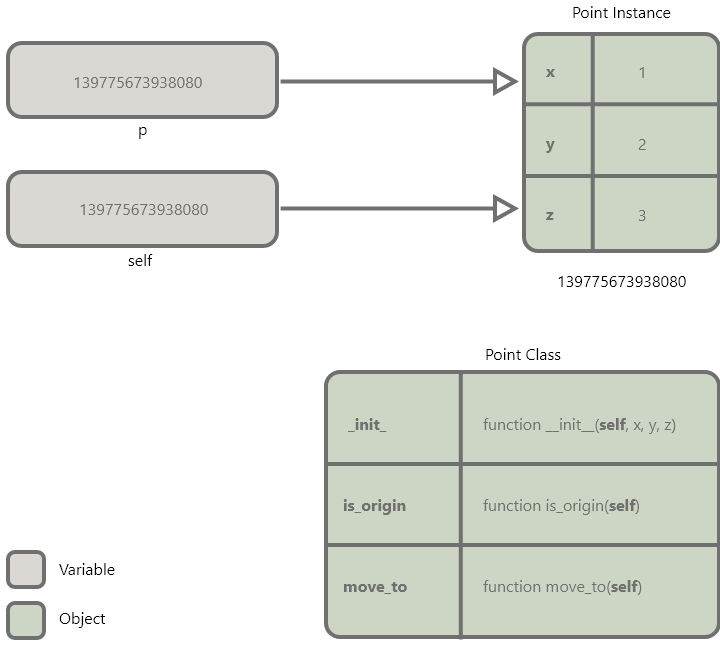Get one quick Python tip each week Weekly Python advice: one quick Python tip every week

# Python's self

Share
Series: Classes3 min. read Watch as video Python 3.8—3.11Watch as video
03:28

When you define a class in Python, you'll see `self` everywhere. What is `self` and why is it everywhere?

## A Python class with `self` in each method

The below `Point` has three methods: a `__init__` method, an `is_origin` method, and a `move_to` method.

``````class Point:

def __init__(self, x, y, z):
self.move_to(x, y, z)

def is_origin(self):
return self.x == self.y == self.z == 0

def move_to(self, x, y, z):
self.x, self.y, self.z = x, y, z
``````

We can make an instance of this class, by calling it. We can access attributes and can call methods on this class instance:

``````>>> p = Point(1, 2, 3)
>>> p.x
1
>>> p.is_origin()
False
>>>
``````

## Do we need that `self` argument?

Each of the methods in our `Point` class accepts `self` as its first argument. What do you think will happen, if we delete that `self` argument?

``````class Point:

def __init__(x, y, z):
self.move_to(x, y, z)

def is_origin():
return self.x == self.y == self.z == 0

def move_to(x, y, z):
self.x, self.y, self.z = x, y, z
``````

Now when we call the `Point` class to make a new `Point` object, we'll see an error:

``````>>> p = Point(1, 2, 3)
Traceback (most recent call last):
File "<stdin>", line 1, in <module>
TypeError: __init__() takes 3 positional arguments but 4 were given
>>>
``````

The error says `__init__` (our initializer method) takes three positional arguments, but four were given. We only passed three arguments into our `Point` class; it got four arguments because `self` was passed in as the first argument (before the three we specified).

So whether you like it or not, the first argument to every one of your methods is going to be `self`, which means we need to capture this `self` thing that's passed in as the first argument.

## What is `self`?

So what is `self`?

Let's temporarily change our `is_origin` method here to return the `id` of `self`:

``````    def is_origin(self):
return id(self)
``````

Python's `id` function returns a number representing the memory location of a particular object.

If we call the `is_origin` function, we get a number.

``````>>> p = Point(1, 2, 3)
>>> p.is_origin()
139775673938080
``````

If we look at the `id` of the `p` variable we made, we're going to get the same number:

``````>>> id(p)
139775673938080
``````

That variable `p` points to a `Point` object (remember variables are pointers in Python). That `self` variable in our method call points to the same exact object.

So `self` is really just a variable that points to the instance of our class that we're currently working with.## But what does `self` mean? Could we rename it?

What if we take `self` everywhere in the code and change it to `this`?

``````class Point:

def __init__(this, x, y, z):
this.move_to(x, y, z)

def is_origin(this):
return this.x == this.y == this.z == 0

def move_to(this, x, y, z):
this.x, this.y, this.z = x, y, z
``````

Would our code still work?

If we make a new `Point` object again, we'll see that we everything works as it did before: we can still access attributes and we can still call methods:

``````>>> p = Point(1, 2, 3)
>>> p.x
1
>>> p.is_origin()
False
``````

From Python's perspective, it doesn't actually matter, what you call `self`. You just have to accept that the instance of your class will be passed in as the first argument. The `self` variable is just a very strong convention, you should call it `self` (otherwise other Python programmers will be confused when reading your code) but you're allowed to call it something else.

## `self` is the first argument of every method in Python

When Python calls a method in your class, it will pass in the actual instance of that class that you're working with as the first argument. Some programming languages use the word `this` to represent that instance, but in Python we use the word `self`.

When you define a class in Python, every method that you define, must accept that instance as its first argument (called `self` by convention).

The `self` variable points to the instance of the class that you're working with.

#### Series: Classes

Classes are a way to bundle functionality and state together. The terms "type" and "class" are interchangeable: `list`, `dict`, `tuple`, `int`, `str`, `set`, and `bool` are all classes.

You'll certainly use quite a few classes in Python (remember types are classes) but you may not need to create your own often.